Courses

# Test: Velocity & Acceleration Analysis - 3

## 10 Questions MCQ Test Theory of Machines (TOM) | Test: Velocity & Acceleration Analysis - 3

Description
This mock test of Test: Velocity & Acceleration Analysis - 3 for Mechanical Engineering helps you for every Mechanical Engineering entrance exam. This contains 10 Multiple Choice Questions for Mechanical Engineering Test: Velocity & Acceleration Analysis - 3 (mcq) to study with solutions a complete question bank. The solved questions answers in this Test: Velocity & Acceleration Analysis - 3 quiz give you a good mix of easy questions and tough questions. Mechanical Engineering students definitely take this Test: Velocity & Acceleration Analysis - 3 exercise for a better result in the exam. You can find other Test: Velocity & Acceleration Analysis - 3 extra questions, long questions & short questions for Mechanical Engineering on EduRev as well by searching above.
QUESTION: 1

### Consider a four-bar mechanism shown in the given figure. The driving link DA is rotation uniformly at a speed of 100 rpm clockwise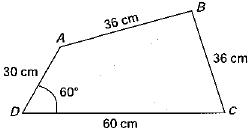Q. The velocity of A will be

Solution: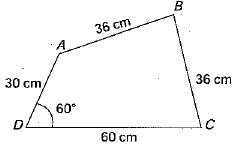V = rωQUESTION: 2

### The directions of Coriolis component of acceleration, 2 ωV, of the slider A with respect to the coincident point B is shown in figure 1,2, 3 and 4. Directions shown by figures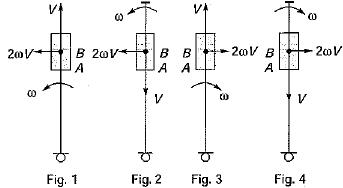Solution:

fcor = 2Vω
2 and 4 are wrong according to above equation. These should be as show below.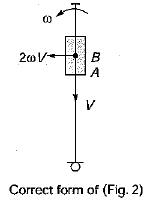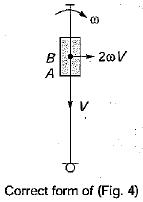QUESTION: 3

### In the figure shown, the relative velocity of link 1 with respect of link 2 is 12 m/sec. Link 2 rotates at a constant speed of 120 rpm. The magnitude of Coriolis component of acceleration of link 1 is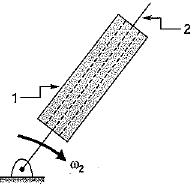Solution:

Velocity of link 1 with respect to 2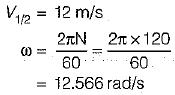∴ Coriolis component of acceleration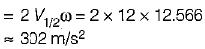QUESTION: 4

The number of links in a planer mechanism with revolute joints having 10 instantaneous centre is

Solution:

Number of instantaneous centre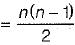for n = 5
we get Number of instantaneous centre = 10

QUESTION: 5

The figure below shows a planer mechanism with single degree of freedom. The instant center 24 for the given configuration in located at a position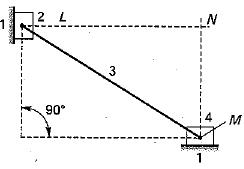Solution:
QUESTION: 6

Figure shows a quick return mechanism. The crank OA rotates clockwise uniformly.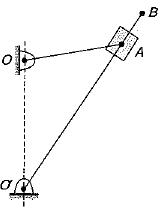OA = 2 cm; OO' = 4 cm
Q. The ratio of time for forward motion to that for return motion is

Solution: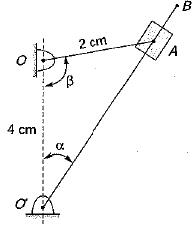sin α = 2/4
α = 30°
∴ β = 60°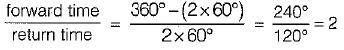QUESTION: 7

A slider sliding at 10 cm/s on a link which is rotating at 60 rpm is subjected to Coriolis acceleration of magnitude

Solution:

Coriolis acceleration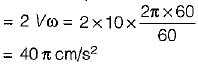QUESTION: 8

In the figure given the link 2 rotates at an angular velocity of 2 rad/s. What is the magnitude of Coriolis acceleration experienced by the link 4?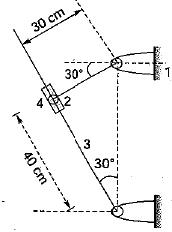Solution:

ω = 0
∴ fcor = 0

QUESTION: 9

A simple quick return mechanism is shown in the figure. The forward to return ratio of the quick return mechanism is 2 : 1. If the radius of the crank O1P is 125 mm, then the distance ‘of’ (in mm) between the crank centre to lever pivot centre point should be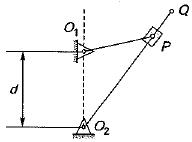Solution: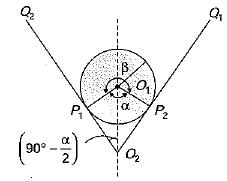O1P = 125mm
Quick return mechanism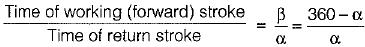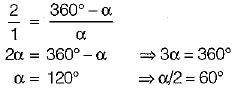The extreme position of the crank (O1P) are shown in figure.
From right angle triangle O2O1P1, we find that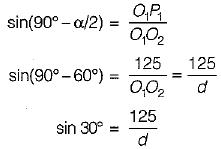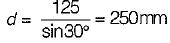QUESTION: 10

For the configuration shown, the angular velocity of link AB is 10 rad/s counter clockwise. The magnitude of the relative sliding velocity (in ms-1) of slider B with respect to rigid link CD is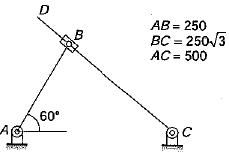Solution:

As AB is perpendicular to slider for given condition, so sliding velocity of slider equals to velocity of link AB
= ω x AB = 10 x 0.25
= 2.5 m/s